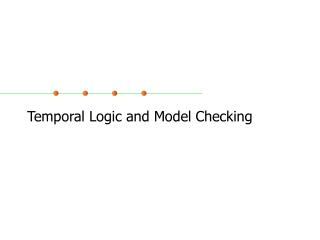Download PresentationTemporal Logic and Model Checking

# Temporal Logic and Model Checking - PowerPoint PPT PresentationDownload Presentation## Temporal Logic and Model Checking

- - - - - - - - - - - - - - - - - - - - - - - - - - - E N D - - - - - - - - - - - - - - - - - - - - - - - - - - -
##### Presentation Transcript

1. Temporal Logic and Model Checking

2. Reactive Systems • We often classify systems into two types: • Transformational: functions from inputs available at the start of the computation to outputs provided on termination • Reactive: behaviour is in general infinite: a process in a reactive system is usually non terminating continuously responding to stimuli from its environment • Implication – these different systems need different techniques for reasoning about system correctness

3. Reasoning about Correctness • A system state is a snapshot of the system’s variables at some point in time • System changes state in response to stimuli • A pair of states, one before action, one after is called a transition • The computation of a reactive system is a possibly infinite sequence of states, each obtained from the previous state via a transition • Clarke slides • http://www-2.cs.cmu.edu/~emc/15-820A/reading/lecture_0.pdf

4. Start Close Heat Error Start Close Heat Error Start Close Heat Error Start Close Heat Error Start Close Heat Error Start Close Heat Error Start Close Heat Error start oven close door open door open door cook done start cooking open door close door reset start oven warmup Example: Microwave Oven

5. CTL Specification • We would like the microwave to have the following properties (among others): • No heat while door is open • AG( Heat→Close): • If oven starts, it will eventually start cooking • AG (Start→ AFHeat) • It must be possible to correct errors • AG( Error→AF ¬ Error): • Does it? How do we prove it?

6. Model Checking Problem • Given a Kripke structure M = (S,R,L) that represents a finite-state transition graph and a temporal logic formula f • Find all states in S that satisfy f: • {s  S | M,s ╞ f } • and check that initial states are among these.

7. CTL Model Checking Algorithm • Iterate over subformulas of f from smallest to largest • For each s  S, if subformula is true in s, add it to labels(s) • When algorithm terminates • M,s ╞ f iff f  labels(s)

8. Checking Subformulas • Any CTL formula can be expressed in terms of: ¬, , EX, EU, and EG, • Therefore must consider 6 cases: • Atomic proposition • if ap  L(s), add to labels(s) • ¬f1 • if f1labels(s), add ¬f1to labels(s) • f1 f2 • if f1labels(s) or f1labels(s), add f1 f2 tolabels(s) • EX f1 • add EX f1 to labels(s) if successor of s, s', has f1labels(s')

9. Checking Subformulas • E[f1 U f2] • Find all states s for which f2  labels(s) • Follow paths backwards from s finding all states that can reach s on a path in which every state is labeled with f1 • Label each of these states with E[f1 U f2]

10. Checking Subformulas • EGf1 • Basic idea – look for one infinite path on which f1 holds. • Decompose M into nontrivial strongly connected components • A strongly connected component (SCC) C is • a maximal subgraph such that every node in C is reachable by every other node in C on a directed path that contained entirely within C. • C is nontrivial iff either • it has more than one node or • it contains one node with a self loop • Create M' = (S’,R’,L’) from M by removing all states s  S in which f1labels(s) and updating S, R, and L accordingly

11. Checking Subformulas • Lemma M,s ╞ EGf1 iff • s  S' • There exists a path in M' that leads from s to some node t in a nontrivial strongly connected component of the graph (S', R'). • Proof left as exercise, but basic idea is • Can’t have an infinite path over finite states without cycles • So if we find a path from s to a cycle and f1 holds in every state (by construction) • Then we’ve found an infinite path over which f1 holds

12. Checking EF f1 procedure CheckEG(f1) S' = {s |f1labels(s)}; SCC = {C| C is a nontrivial SCC of S'}; T = C  SCC{s | s  C}; for all s Tdolabels(s) = labels(s)  {EG f1}; while T ≠  do choose s T; T = T \ {s}; for all tsuch that t  S' and R(t,s) do if EG f1 labels(t) then labels(t) = labels(t)  {EG f1}; T = T {t}; end if; end for all; end while; end procedure;

13. Checking a Property • Checking AG(Start → AF Heat) • Rewrite as ¬EF(Start  EG ¬Heat) • Rewrite as ¬ E[ true U (Start  EG ¬Heat)] • Compute labels for smallest subformulas • Start, Heat • ¬ Heat

14. Checking a Property • Compute labels for EG ¬Heat • S’ = {1,2,3,5,6} • SCC = {{1,2,3,5}} • T = {1,2,3,5} • No other state in S’ can reach a state in T along a path in S’. • Computation terminates. States 1,2,3, and 5 labelled with EG ¬Heat

15. Checking a Property • Compute labels for Start EG¬Heat

16. Checking a Property • E[true U(Start EG¬Heat)] • Start with set of states in which Start EG¬Heat holds i.e., {2,5} • Work backwards marking every state in whichtrue holds

17. Checking a Property • Check ¬ E[true U(Start  EG ¬Heat)] • Leaves us with the empty set, so safety property doesn’t hold over microwave oven